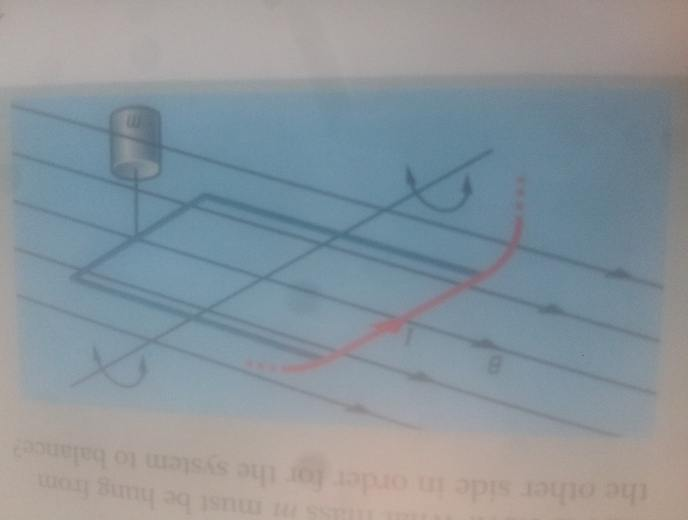# How does a current balance work?

• Tangeton

#### Tangeton

< Mentor Note -- thread moved to HH from the technical physics forums, so no HH Template is shown >

I am looking for a very simplistic and understandable explanation for how it works as I don't seem to find any place that explains it well... I am just doing A Level which is equivalent to high school.

Also I've looked at a practice question in the book, and I've been given B (2.0x10^-3) and current (1.0A). I've been asked to find what the mass must be hanged on the other side in order for the system to be balanced, but I don't know how to since I haven't been given the length of the wire... I wanted to equate F = BIL (if I had the length) to the weight of the mass (mg) but I cannot do that unless I assume some sort of length. When I said l = 1 then I got 2.0 x 10^-4. There is no answer in the book... any ideas of how I'd approach this?

Last edited by a moderator:

< Mentor Note -- thread moved to HH from the technical physics forums, so no HH Template is shown >

I am looking for a very simplistic and understandable explanation for how it works as I don't seem to find any place that explains it well... I am just doing A Level which is equivalent to high school.

Also I've looked at a practice question in the book, and I've been given B (2.0x10^-3) and current (1.0A). I've been asked to find what the mass must be hanged on the other side in order for the system to be balanced, but I don't know how to since I haven't been given the length of the wire... I wanted to equate F = BIL (if I had the length) to the weight of the mass (mg) but I cannot do that unless I assume some sort of length. When I said l = 1 then I got 2.0 x 10^-4. There is no answer in the book... any ideas of how I'd approach this?

What's a current balance? Can you post a diagram or picture?

Also, it's best if you make a habit of carrying units along in your variables and calculations. When you say you are given "given B (2.0x10^-3)", what are the units?

What's a current balance? Can you post a diagram or picture?

Also, it's best if you make a habit of carrying units along in your variables and calculations. When you say you are given "given B (2.0x10^-3)", what are the units?

Unit is the Tesla.